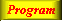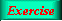Lecture 8 - Page 5 : 11
 Functional Programming in SchemeObject-oriented programming in Scheme * Closures revisited Functions and closures * Classes and objects in Scheme Functions with private context Classes and objects A general pattern of classes Example of the general class pattern A general pattern of classes with inheritance An example of classes with inheritance The interpretation of self A demo of virtual methods
 Classes and objects
 Due to (1) the first class status of functions, and due to (2) the use of static binding of free names, it is possible to interpret a closure as an object With this interpretation, it is possible to regard certain function definitions as classesThe send method which is used in the Point class.

The function apply calls a function on a list of parameters. This should be seen in contrast to a normal call, in which the individual parameters are passed.

 ```(define (point x y) (letrec ((getx (lambda () x)) (gety (lambda () y)) (add (lambda (p) (point (+ x (send 'getx p)) (+ y (send 'gety p))))) (type-of (lambda () 'point)) ) (lambda (message) (cond ((eq? message 'getx) getx) ((eq? message 'gety) gety) ((eq? message 'add) add) ((eq? message 'type-of) type-of) (else (error "Message not understood"))))))```

The definition of a 'class Point ' with methods getx , gety , add , and type-of . On this page we have also defined the syntactical convenience function send that sends a message to an object. In MzScheme, be sure that you define send before Point (such that send in the add method refers to our send , and not an already existing and unrelated definition of the name send ).All necessary stuff to play with Point.Points and Rectangle